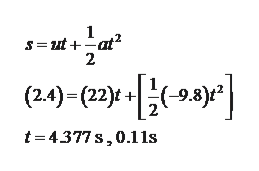A golf ball is struck with an initial velocity of 22 m/s at an angle of 23 degrees above the horizontal. On its downward trajectory, the ball hits an elevated green 2.4 meters above the tee elevation. Determine the flight time in seconds.Determine the horizontal distance traveled (meters) by the golf ball under the conditions described above.Determine the magnitude of the golf ball velocity (m/s) when it first strikes the green under the conditions described above.

Question

A golf ball is struck with an initial velocity of 22 m/s at an angle of 23 degrees above the horizontal. On its downward trajectory, the ball hits an elevated green 2.4 meters above the tee elevation. Determine the flight time in seconds.

Determine the horizontal distance traveled (meters) by the golf ball under the conditions described above.

Determine the magnitude of the golf ball velocity (m/s) when it first strikes the green under the conditions described above.

Step 1

a)

The required time to flight from kinematics equation is,help_outlineImage Transcriptionclose1 S=Dut + -at고 2 -9.8)* (2.4)- (22)t t 4377s,0.11s fullscreen
Step 2

Since, the time is taken when the ball hits during downward trajectory. Thus, the required time to flight is 4.377s.

b)

The required horizontal travelled distance is,

Step 3

c)

The vertical velocity when the...

Want to see the full answer?

See Solution

Want to see this answer and more?

Our solutions are written by experts, many with advanced degrees, and available 24/7

See Solution
Tagged in

Mechanical Properties of Matter